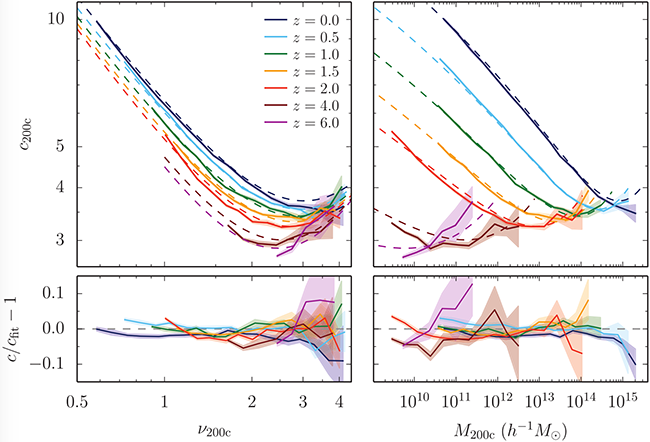### Universal models for the concentration-mass relation of dark matter halos

Concentration is defined as the ratio of an outer radius to the scale radius, e.g., c200c = R200c / rs. An NFW profile can be parameterized by only a mass (or radius) and concentration, making the concentration-mass relation an important ingredient in modeling the density profiles of halos when only their mass is known.

Traditionally, the c-M relation has been described in two ways. First, concentration is tightly related to halo age, meaning that a prediction for the age of halos as a function of mass can be turned into a prediction for concentration (e.g., Wechsler et al. 2002). Second, the c-M relation is more or less a power law at fixed redshift and cosmology, leading to numerous numerical calibrations (e.g., Dutton & Maccio 2014).

There is, however, a third way to model concentrations: by considering the properties of the density peaks they originated from. Concentration is almost universal (i.e., independent of redshift) when expressed in units of peak height rather than mass (e.g., Prada et al. 2012). This is demonstrated in the figure below: the redshift evolution of the concentration-peak height relation (left) is much less dramatic than that of the concentration-mass relation (right).In Diemer & Kravtsov 2015 (DK15, see details below), we showed that the residual non-universality is due to the shape of the power spectrum, and built a model that is a function of only peak height and the logarithmic power spectrum slope (dashed lines in the figure above). With only seven free parameters, this model matches our ΛCDM results (for different cosmologies) to 5% accuracy up to z = 6, and matches scale-free Ωm=1 models to 15% accuracy. The model also reproduces the low concentrations of Earth-mass halos at z = 30, meaning it extrapolates correctly over 16 orders of magnitude in halo mass. According to our model, there is no well-defined floor in the concentration values. Instead, the minimum concentration depends on redshift: at fixed peak height, halos at higher redshift experience steeper power spectrum slopes, and thus have lower minimum concentrations.

In Diemer & Joyce 2019 (DJ19, see details below), we extended this concept with two key insights. First, at the low-mass end, halos largely grow via pseudo-evolution, meaning that their profile remains more or less constant while their radius and mass grow due to the decreasing density threshold used to define the boundary. Based on self-similar universes, we describe the pseudo-evolution of concentration analytically and thus derive an expression for the low-mass end slope of the c-M relation. Second, the late-time evolution of concentration appears to be influenced by a parameter other than the slope of the power spectrum. We parameterize this extra evolution based on the logarithmic derivative of the growth factor. Due to the semi-analytical nature of the model, it has only six free parameters and fits the data even better than the original DK15 model.

Both the DK15 and DJ19 models can be evaluated using the python code Colossus. Alternatively, we provide tabulated concentrations for a number of cosmologies in the data section.

Related publications:

• Diemer & Joyce
An accurate physical model for halo concentrations
[ads] [arXiv] [2019 ApJ 871, 168]
• Diemer
COLOSSUS: A python toolkit for cosmology, large-scale structure, and dark matter halos
[ads] [arXiv] [2018 ApJS 239, 35]
• Diemer & Kravtsov
A universal model for halo concentrations
[ads] [arXiv] [2015 ApJ 799, 108]
• Diemer & Kravtsov
Poster: A universal model for halo concentrations
[pdf]